•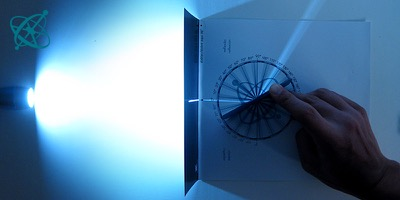Can you predict the direction of the reflected light?

•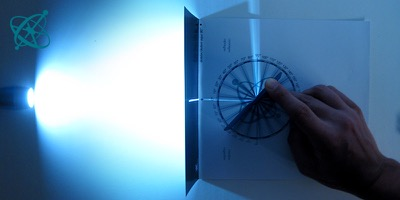What law is governing reflections?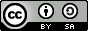www.sciensation.org | Ciênsação hands-on experiments are published as Open Educational resources under a Creative Commons Attribution-ShareAlike 4.0 International License.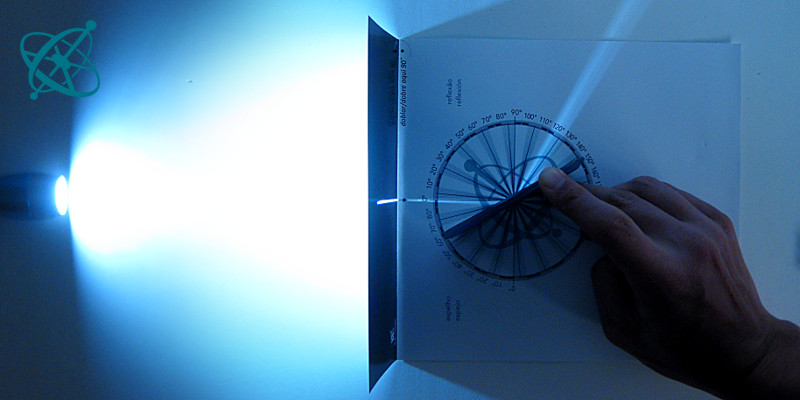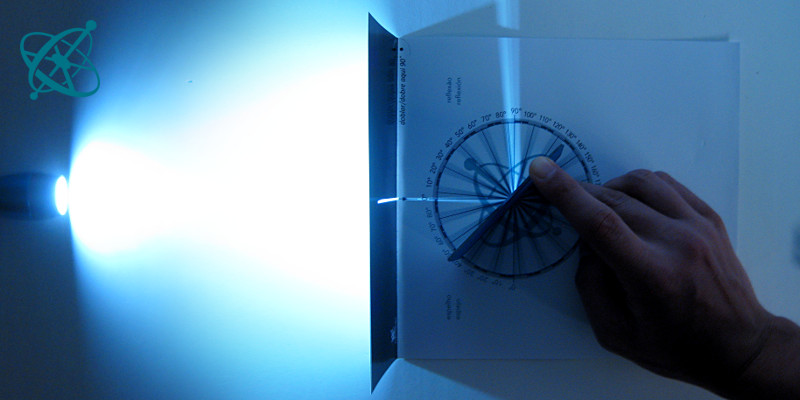Physics
age: 8 – 12

# The Law of Reflection

Let your students discover the law of reflection. Of course, you could teach this fact with a single sentence. But then you would miss a nice opportunity: Precisely because it is so simple, your students will figure it out in a few minutes and hone their scientific skills.

Learning objective

The angle of incidence equals the angle of reflection.

Finding a natural 'law' in the process of experimenting, reasoning and formulating of concise scientific statements.

Printout of the worksheet
Mirror
Torch (or similar light source)
Scissors
Preparation

Print out this worksheet for each group of students and hand it out together with torches and mirrors.

Fold the worksheet and cut out a slit where the black box is marked with a white line. Then shine the light of a torch through the slit along the line pointing towards the center of the circle. Hold a mirror in the center with its reflective surface towards the slit and start rotating it clockwise.

1. Describe in a few words how to predict the direction in which the mirror reflects the light.

Guiding Questions (if needed)

What do you need to know in order to predict the direction that the reflected light will take?
› Under what angle the light hits the mirror.

Which angles do the angular scales show?
› The left one shows the rotation of the mirror, while the right one shows the angle between the incoming and the reflected light.

Is there a better way to define the angles, so that your formulation becomes more concise?
› One can define the angles relative to the mirror plane or its normal.

Findings

If the mirror is slightly tilted forward, the reflected light can be seen on the angular scale. Your students will rapidly find a relation between angle of incident and angle of reflection. In case they claim "it is always the double", you could use the second question in "Guiding Questions" above.

It is worthwhile to insist on an accurate formulation of this relation – which might well differ from the standard "angle of incidence equals angle of reflection". Once this is achieved, let your students know that they have just discovered a scientific law in the same way professional scientists would do.